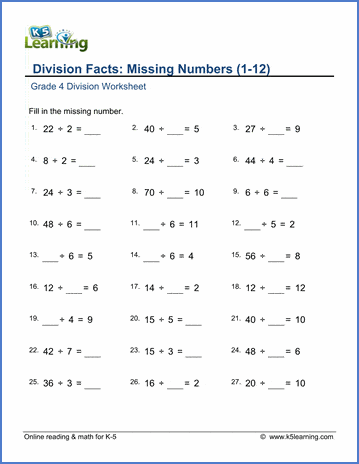# New Grade 4 Math Worksheets Pages

We have reworked our grade 4 math worksheets, and added a number of new worksheets. Our worksheets now include:Mental addition, adding 2-, 3-, 4-, 5- and 6-digit numbers in columns and adding 3-digit numbers in columns.

## Subtraction

Mental subtraction, subtracting 3-, 4-, 5- and 6-digit numbers in columns - with and without borrowing.

## Place value & rounding

Building 4-, 5- and 6-digit numbers from their parts and rounding numbers to the nearest 10, 100, 1,000 and 10,000.

## Mental multiplication

Multiplication tables 2-12, multiplying single-digits by whole tens and hundreds, multiplying whole tens, hundreds and thousands and multiplying in parts.

## Multiplying in columns

Single-digit and double-digit multiplication.

## Mental division

Division facts practice (1-12), dividing by 10 and 100, dividing by whole tens and hundreds, division with remainder.## Long division

Dividing 2-, 3-, and 4-digits by 1-digit numbers - with, and with no, remainder.

## Factoring

Finding the factors of a number between 4-50, and 4-100.

## Fractions

Adding and subtracting fractions, converting fractions to/from mixed numbers, comparing fractions, equivalent fractions and converting fractions to/from decimals.

## DecimalsAdding and subtracting decimal numbers.

## Roman numerals

Writing Roman numerals as normal numbers, and writing normal numbers as Roman numerals.

## Geometry

Classifying angles, triangle and quadrilaterals, finding the are and perimeters of rectangles, finding the circumference of a circle and plotting points on coordinate grids.

## Measurement

Converting customary units and metric units.

## Math word problems

Multiplication and division word problems, fractions and decimals word problems.